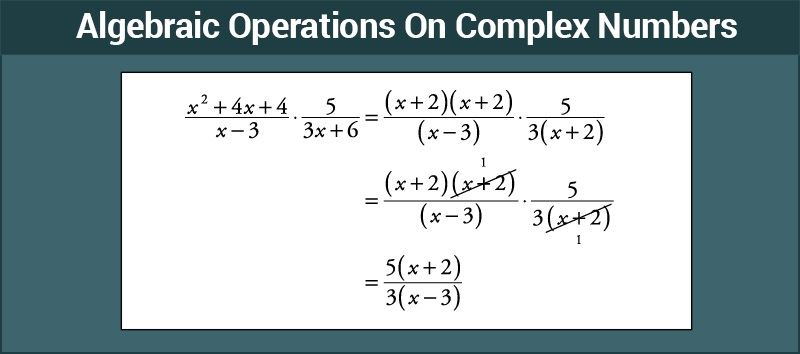# Algebraic Operations On Complex Numbers

In Mathematics, algebraic operations are similar to the basic arithmetic operations which include addition, subtraction, multiplication, and division. The algebraic operations are defined purely by the algebraic methods. Some basic algebraic laws like associative, commutative, and distributive law are used to explain the relationship between the number of operations. By the use of these laws, the algebraic expressions are solved in a simple way. Since algebra is a concept based on known and unknown values (variables), the own rules are created to solve the problems.  In this article, let us discuss the basic algebraic operations on complex numbers with examples.

## What are Complex Numbers?

In Maths, basically, a complex number is defined as the combination of a real number and an imaginary number. The real numbers are the numbers which we usually work on to do the mathematical calculations. But the imaginary numbers are not generally used for calculations but only in the case of complex numbers.## Operations on Complex Numbers

The basic algebraic operations on complex numbers discussed here are:

• Addition of Two Complex Numbers
• Subtraction(Difference) of Two Complex Numbers
• Multiplication of Two Complex Numbers
• Division of Two Complex Numbers.

### Addition of Two Complex Numbers

We know that a complex number is of the form z=a+ib where a and b are real numbers.

Consider two complex numbers z1 = a1 + ib1 and z2 = a2 + ib2

Then the addition of the complex numbers z1 and z2 is defined as,

z1+z2 =( a1+a2 )+i( b1+b2 )

We can see that the real part of the resulting complex number is the sum of the real part of each complex numbers and the imaginary part of the resulting complex number is equal to the sum of the imaginary part of each complex numbers.

That is, Re(z1+z2 )= Re( z1 )+Re( z1 )

Im( z1+z2 )=Im( z1)+Im(z2)

For the complex numbers,

z1 = a1+ib1

z2 = a1+ib2

z3 = a3+ib3

………..

………..

zn = an+ibn

a1+a2+a3+….+an = (a1+a2+a3+….+an )+i(b1+b2+b3+….+bn)

 Let’s Work Out: Example: z1 = a+3i, z2 = 4+bi, z3 = 6+10i Find the value of a and b if z3 = z1+z2 Solution: By the definition of addition of two complex numbers, Re(z3 ) = Re(z1 )+Re(z2 ) 6 = a + 4 a = 6 – 4 = 2 Im(z3 ) = Im(z1 ) + Im(z2 ) 10 = 3+b b = 10-3 =7

Note: Conjugate of a complex number z=a+ib is given by changing the sign of the imaginary part of z which is denoted as $\bar z$

$\bar z = a-ib$

$z+ \bar z$ =2a

$z- \bar z$ =2bi

### Difference of Two Complex Numbers

Consider the complex numbers z1 = a1+ib1 and z2 = a2+ib2, then the difference of z1 and z2, z1-z2 is defined as,

z1-z2 = (a1-a2)+i(b1-b2)

From the definition, it is understood that,

Re(z1-z2)=Re(z1)-Re(2)

Im(z1-z2)=Im(z1)-ImRe(2)

 Example: z1 =4+ai,z2=2+4i,z3 =2. Find the value of a if z3=z1-z2 Solution: By the definition of difference of two complex numbers, Im3=Im1-Im2 0 = a – 4 a = 4

Note: All real numbers are complex numbers with imaginary part as zero.

### Multiplication of Two Complex Numbers

We know the expansion of (a+b)(c+d)=ac+ad+bc+bd

Similarly, consider the complex numbers z1 = a1+ib1 and z2 = a2+ib2

Then, the product of z1 and z2 is defined as

z1 z2=(a1+ib1)(a2+ib2)

$z_1 z_2 = a_1 a_2+a_1 b_2 i+b_1 a_2 i+b_1 b_2 i^2$

Since, $i^2$=-1,

$z_1 z_2 = (a_1 a_2-b_1 b_2 )+i(a_1 b_2+a_2 b_1 )$

 Example: z1=6-2i, z2=4+3i. Find z1 z2 Solution: z1 z2 = (6-2i) (4+3i) = 6 × 4 + 6 × 3i + (-2i) × 4 + (-2i)(3i) = 24 + 18i – 8i – 6i = 24 + 10i + 6 = 30 + 10i

Note: Multiplicative inverse of a complex number

Definition: For any non-zero complex number z=a+ib(a≠0 and b≠0) there exists a another complex number $z^{-1} ~or~ \frac {1}{z}$ which is known as the multiplicative inverse of z such that $zz^{-1} = 1$.

z = a+ib, then $z^{-1} = \frac{a}{a^2 + b^2} + i \frac{(-b)}{a^2 + b^2}$

$Re(z^{-1}) = \frac{a}{a^2 + b^2}$

$Im(z^{-1}) = \frac{-b}{a^2 + b^2}$

 Example: z = 3 + 4i Solution: $z^{-1}$ of $a + ib$ = $\frac{a}{a^2 + b^2} +i \frac{(-b)}{a^2 + b^2}$ = $\frac{(a-ib)}{a^2 + b^2}$ Numerator of $z^{-1}$ is conjugate of z, that is a – ib Denominator of $z^{-1}$ is sum of squares of the Real part and imaginary part of z Here, $z$ = $3 + 4i$ $z^{-1}$ = $\frac{3-4i}{3^2 + 4^2}$ = $\frac{3-4i}{25}$ $z^{-1}$ = $\frac{3}{25} – \frac{4i}{25}$

### Division of Complex Numbers

Consider the complex number $z_1$ = $a_1 + ib_1$ and $z_2$ =$a_2 + ib_2$, then the quotient ${z_1}{z_2}$ is defined as,

$\frac{z_1}{z_2}$ = $z_1 × \frac{1}{z_2}$

Therefore, to find $\frac{z_1}{z_2}$ , we have to multiply $z_1$ with the multiplicative inverse of $z_2$.

 Example: $z_1$ = $2 + 3i$ and $z_2$ = $1 + i$, Find $\frac{z_1}{z_2}$. Solution: $\frac{z_1}{z_2}$ = $z_1 × \frac{1}{z_2}$ $\frac{2+3i}{1+i}$ = $(2+3i) × \frac{1}{1+i}$ ∵ $\frac{1}{1+i}$ = $\frac{1-i}{1^2 + 1^2}$ = $\frac{1-i}{2}$ $\frac{2 + 3i}{1 + i}$ = $2+3i × \frac{1-i}{2}$= $\frac{(2+3i)(1-i)}{2}$ =$\frac{2 – 2i + 3i – 3i^2}{2}$= $\frac{5+i}{2}$

Here, you have learnt the algebraic operations on complex numbers. There are many more things to be learnt about complex number. Log onto www.byjus.com to cover more topics.